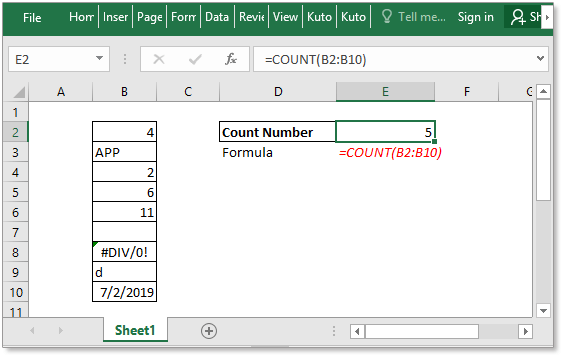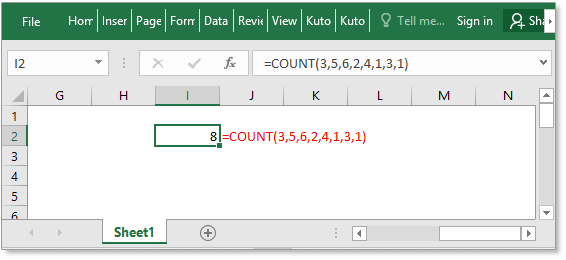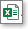## Excel COUNT function### Description

The COUNT function is used to count the number of cells that contain numbers, or count the numbers in a list of arguments. Take an instance, COUNT(1,2,3,4,11,2,1,0) returns 8.

### Syntax and arguments

Formula syntax

 COUNT(value1,[value2], …)

Arguments

 Value1: Required, the first item, cell reference, or range. Value2: Optional, an item, cell reference, or range within which you want to count numbers.

Return Value

The COUNT function returns the number of cells that contains numbers.

Remarks

• The COUNT function counts the number of numbers in supplied arguments, for example, =COUNT(0,1,2,3,”text”) returns 4 since only 4 numbers are in the arguments.
• The COUNT function can count the number of numbers, dates or a text representation of numbers (numbers enclosed with quotation marks, says “8”).
• The arguments are up to 255 additional items.
• The arguments which cannot be translated to numbers will not be counted.
• If the argument is an array or reference, only the number values in the array or reference will be counted.
• Empty cells, logical values, error values or texts will not be counted.

### Usage and Examples

Example 1

There is a list of values in a sheet. Now you can count the numbers in this list with this formula:

 =COUNT(B2:B10)

Press Enter key to count the number of numbers in the range B2:B10.Example 2

Directly type the values that you want to count into the formula as arguments.

 =COUNT(3,5,6,2,4,1,3,1)

Press Enter key to return 8.Click to download COUNT function sample file

#### Relative Functions:

• Excel COUNTA Function
The COUNTA function returns the number of cells that contain number, text, error value or the empty text “”, and excluding the real empty cells.

• Excel COUNTBLANK Function
The COUNTBLANK function returns the number of empty cells. The COUNTBLANK function is a built-in statistical function, which can be used as a worksheet function (WS) in Excel.

• Excel COUNTIF Function
The COUNTIF function is a statistical function in Excel which is used to count the number of cells that meet a criterion.

• Excel COUNTIFS Function
The COUNTIFS function returns the number of cells that meet one single criterion or multiple criteria. The COUNNTIFS function is one of the built-in statistical function, and it can be used as a worksheet function (WS) in Excel.

#### Relative Articles:

• Count cells equal to
If you want to count cells exactly match a given criterion, you can choose the COUNTIF function or the SUMPRODUCT (combine with the EXACT function) function to count cells exactly equal to without case sensitive or with case sensitive.

• Count cells not equal to
To count the number of cells that contain values that not equal to a specified value, such as below screenshot shown. In Excel, you can use the COUNTIF function to quickly handle this job.

• Count cells equal to x or y
If you want to count cells as long as that meet at least one of given conditions (OR logic) in Excel, i.e. count cells equal to either x or y, you can use several COUNTIF formulas or combine the COUNTIF formula and SUM formula to handle this job.

• Count cells equal to x and y
Here in this tutorial, it will provide some formulas to talk about counting cells that match with all given conditions, which we call it the AND logic with the COUNTIFS function.

### The Best Office Productivity Tools

#### Kutools for Excel - Helps You To Stand Out From Crowd

Would you like to complete your daily work quickly and perfectly? Kutools for Excel brings 300 powerful advanced features (Combine workbooks, sum by color, split cell contents, convert date, and so on...) and save 80% time for you.

• Designed for 1500 work scenarios, helps you solve 80% Excel problems.
• Reduce thousands of keyboard and mouse clicks every day, relieve your tired eyes and hands.
• Become an Excel expert in 3 minutes. No longer need to remember any painful formulas and VBA codes.
• 30-day unlimited free trial. 60-day money back guarantee. Free upgrade and support for 2 years.#### Office Tab - Enable Tabbed Reading and Editing in Microsoft Office (include Excel)

• One second to switch between dozens of open documents!
• Reduce hundreds of mouse clicks for you every day, say goodbye to mouse hand.
• Increases your productivity by 50% when viewing and editing multiple documents.
• Brings Efficient Tabs to Office (include Excel), Just Like Chrome, Firefox, And New Internet Explorer.Say something here...
symbols left.